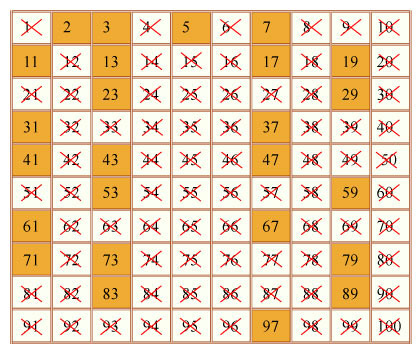# Primes

The reminders about Amazon Prime day got me thinking about Prime Numbers. Whilst I was coding a power efficient “blink” for a new 4Tronix board I joked that it was not calculating primes. But then I thought, “why not?” I remembered back to my ZxSpectrum days and thought, it that can calculate primes then there’s no reason it could not be done on an Arduino. The piece of code I used on the Spectrum was called the Sieve of Eratosthenes. I’m not sure that I understood it properly at the time but it works by taking all of the numbers in an array and removing all the multiples of prime numbers from that array.  I found an example in C and tweaked it to work on the Arduino.``` void setup() { //Based on http://www.programminglogic.com/the-sieve-of-eratosthenes-implemented-in-c/```

#define LIMIT 32700 /*size of integers array*/

byte primes[LIMIT];
unsigned long i,j;
int z = 1;

for (i=2;i<LIMIT;i++)
primes[i]=1;

for (i=2;i<LIMIT;i++)
if (primes[i])
for (j=i;i*j<LIMIT;j++)
primes[i*j]=0;

Serial.begin(9600);

for (i=2;i<LIMIT;i++)
if (primes[i]) {
Serial.print(z++);
Serial.print(“th prime = “);
Serial.println(i);
}
}

``` ```

```void loop() { // this loop is left intentionally blank }```

Let me know if you come up with a faster version or one that can calculate bigger primes.

## Reference

Sieve of Erathoshenes In C